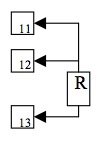Calibrating Green Series Controllers for 10Ω Cu RTD Input

Overview:Figure 1. 10Ω Cu RTD connection ("R" is the resistance leads from the RTD)

Parameter sets of the Green Series controllers are loaded with factory input types including Thermocouples and 100Ω RTDs. Many industrial applications utilize a 10Ω Cu RTD as the temperature sensor of choice. This application note describes the setup and calibration for Green Series controllers for a 10Ω Cu RTD input.

A UM330 controller was used to demonstrate the procedure. The RTD measurement current, IRTD=0.13mA is standard for most Green Series controllers, so the calibration procedure example can be implemented on any green series controller. In this example, the desired PV meaurement range was 0-100°C.

A Temperature-vs.-Resistance table for a 10Ω Cu RTD was used to obtain the resistance values corresponding to the range high and low temperatures respectively. Two temperatures 300°F (= 148.9°C), -40°F (=-40°C), slightly above and below the PV range were selected from the T-vs.-R table. A voltmeter was then used to measure the corresponding voltages at the controller input terminals yielding Vlow = 1.04mV, and Vhi = 2.02mV. From this measurement, appropriate range values of RH=2.02, RL=1.04 were set. The scale high and low values SH, SL were set to the corresponding Celcius temperatures of SH=148.9, SL=-40.0. The controller was tested and found to be accurate for a 0-100°C measurement range with a 10Ω Cu RTD input. If a different PV measurement range is desired, e.g. 150-400°C, the parameters RH,RL,SH,SL must be set to values corresponding to these range endpoints.

Procedure for calibrating a Green Series controller for a measurement range of 0-100°C using a 10Ω Cu RTD

1. Connect the 10Ω Cu RTD to the controller as shown in fig.1
2. Set the parameter IN= 55 ( input type DC –10 to 20mV).
3. Set the parameter SDP = 1.
4. Set the parameters RH=2.02 and RL=1.04.
5. Set the parameters SH=148.9 and SL=-40.0.

Related Products & Solutions

Mobile Field Device Management (FieldMate)

FieldMate 3.0 is a PC based tool that enables you to see intelligent field devices clearly, know their condition in advance, and act with agility throughout maintenance work flow.

UM330 Digital Indicator with Alarms

The UM330 Digital Indicator with Alarms was discontinued on 1/01/2011. The UTAdvanced UM33A is a replacement product.

Panel Indicators

The UM33A provides up to 9 alarms outputs and input correction function (PV bias, Polygonal line approximation, polygonal line bias). Also, 24 VDC sensor power supply is available as an option.

×

Have Questions?

Contact a Yokogawa Expert to learn how we can help you solve your challenges.

Top